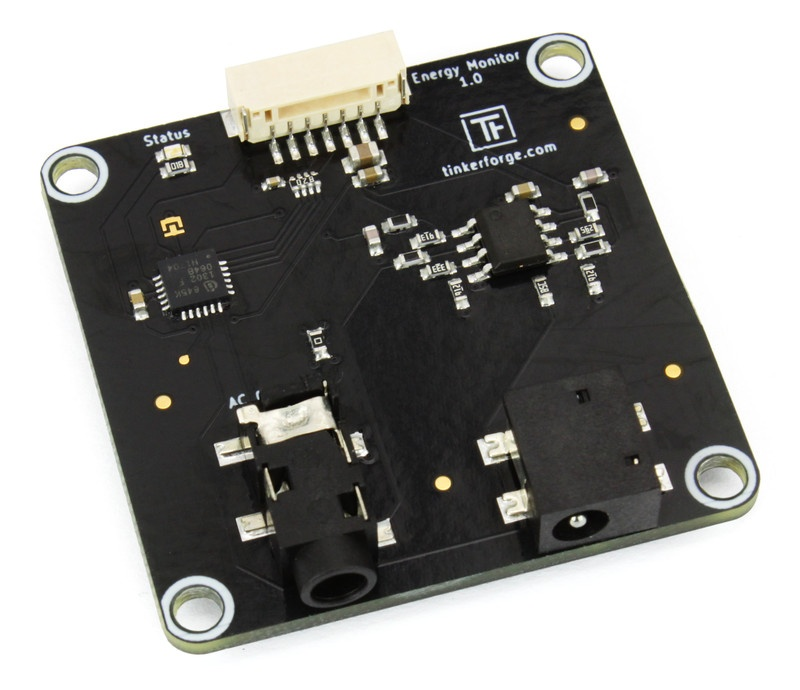# Blog

### Energy Monitor Bricklet

Today we want to introduce the Energy Monitor Bricklet and its features in detail.We will start with the technical specification. The Bricklet can measure the following values:

• Voltage (V): Voltage RMS with a resolution of 10mV
• Current (A): Current RMS with a resolution of 10mA
• Energy (Wh): Energy (integrated over time) with a resolution of 10mWh
• Real Power (W): Real Power with a resolution of 10mW
• Apparent Power (VA): Apparent Power with a resolution of 10mVA
• Reactive Power (VAR): Reactive Power with a resolution of 10mVAR
• Power Factor: Power Factor with a resolution of 1/1000
• Frequency (Hz): AC Frequency of the mains voltage with a resolution of 1/100 Hz
• Waveform Voltage (V): Waveform of the AC Voltage RMS with a resolution of 100mV
• Waveform Current (A):  Waveform of the AC Current RMS with a resolution of 10mV

The waveform is returned as 768 data points. These points correspond to about 3 full sine waves at 50Hz. The Bricklet updates the measured frequency every 6 seconds. All other values are integrated over 10 zero-crossings of the voltage sine wave. With a standard AC mains voltage frequency of 50Hz this results in a 5 measurements per second.

To do these measurements the Energy Monitor Bricklet uses two different transformers:

• AC/AC voltage transformer (230V/9V): This voltage transformer can be plugged into a power outlet (wall socket). It has an input voltage of 230V AC and generates an output voltage of 9V AC. The output voltage is safe and can be measured by the ADC on the Bricklet. All other properties of the voltage sine wave remain the same and can be analyzed accordingly.
• 5A:1V and 30A:1V current transformer clamp (hinged): These current transformers can measure the current that goes through a wire contact-free by transforming it into a voltage (the ratio is 5A/30A to 1V). The output voltage is again safe and can be measured by the ADC on the Bricklet.With both transformers together it is possible to measure the AC voltage and the AC current in a time curve waveform. These measurements in turn can be used to calculate all of the data described above. All of this is possible while there is no live mains voltage anywhere on the Bricklet. The Bricklet can be touched without any problems at any time.

The following video shows a test of the Energy Monitor Bricklet together with four different loads (fan, heater, LED and energy saving light bulb):